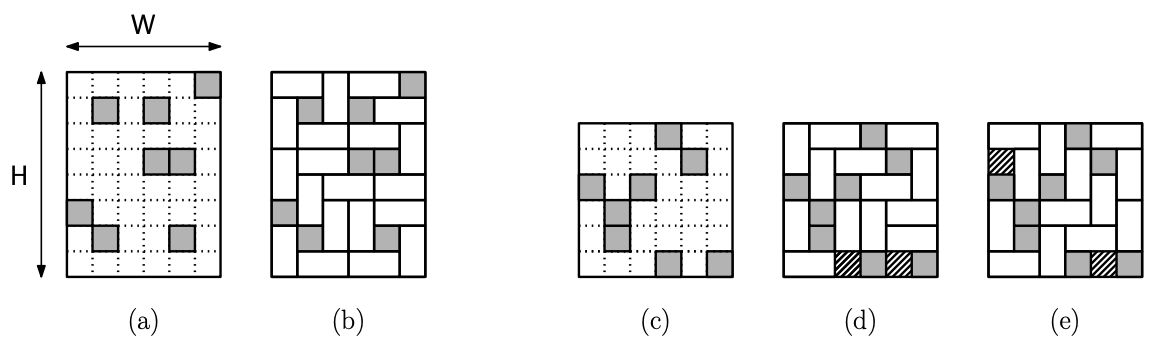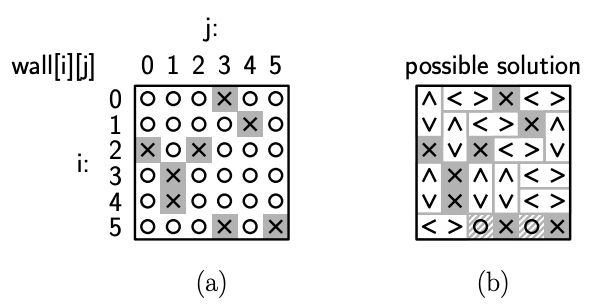시간 제한 메모리 제한 제출 정답 맞은 사람 정답 비율
1 초 256 MB 0 0 0 0.000%

## 문제

Because of major damage to his laboratory (from an explosion involving fart-generating weaponry), Gru needs his minions to replace a large brick wall in their lab. The wall is of size H × W, that is, H squares high and W squares wide (both positive integers). Each brick is of size 1 × 2 and may either horizontally oriented (1 × 2) or vertically oriented (2 × 1). The brick-laying process is complicated by the fact that there are certain squares, called forbidden squares, that must not be bricked over. The minions’ job is to determine a pattern of bricks in order to cover as many of the non-forbidden squares as possible. (Fig. 1(a) shows an example of a wall with forbidden squares shaded, and Fig. 1(b) shows one possible solution for this input.)Figure 1: Sample input walls ((a) and (c)) with forbidden squares shaded, and possible brick layouts ((b), (d), and (e)) with uncovered squares shown with hatched lines.

Note that it may not always be possible to cover all the non-forbidden squares. (You can convince yourself that no pattern of bricks can cover all the squares in Fig. 1(c). In Fig. 1(d) and (e) we show two optimal solutions, each of which leaves two squares uncovered.)

The minions are given as input a two dimensional array of characters, wall, with H rows and W columns (where 1 ≤ H, W ≤ 100). The entry ‘X’ (upper-case ‘X’) means that the square is forbidden and ‘O’ (upper-case ‘O’) means that the square can be covered.Figure 2: (a): Sample input array corresponding Fig. 1(c). (b): sample output array corresponding to the solution of Fig. 1(d).

The output is written into the same array. Each horizontally oriented brick is indicated with two horizontally adjacent array entries ‘’. Each vertically oriented brick is indicated with two vertically adjacent array entries ‘^’ (a caret or Shift-6 on most keyboards) and ‘v’ (lower-case ‘v’). The output is considered correct if it has the proper format and covers the maximum possible number of non-forbidden squares.

## 입력

The first line of the input file contains the number of test cases. Each case has the following format:

• A line containing the height H and width W of the wall array.
• A sequence of H lines, each of containing W characters. Each character is either ‘X’ (forbidden) or ‘O’ (coverable).

You may assume that the input is valid and H and W each lie between 1 and 100.

## 출력

You do not need to generate any output. We will provide a main program that will check that the array that your function returns is of the proper form (although it does not check for optimality), and if so, it will print the tiling layout. Here is an example corresponding to Fig. 2(a) and (b).

## 예제 입력 1

1
6 6
OOOXOO
OOOOXO
XOXOOO
OXOOOO
OXOOOO
OOOXOX


## 예제 출력 1

Case: 1
+---+---+---+---+---+---+
| O | O | O | X | O | O |
+---+---+---+---+---+---+
| O | O | O | O | X | O |
+---+---+---+---+---+---+
| X | O | X | O | O | O |
+---+---+---+---+---+---+
| O | X | O | O | O | O |
+---+---+---+---+---+---+
| O | X | O | O | O | O |
+---+---+---+---+---+---+
| O | O | O | X | O | X |
+---+---+---+---+---+---+

Solution:
+---+---+---+---+---+---+
|   |       | X |       |
+   +---+---+---+---+---+
|   |   |       | X |   |
+---+   +---+---+---+   +
| X |   | X |       |   |
+---+---+---+---+---+---+
|   | X |   |   |       |
+   +---+   +   +---+---+
|   | X |   |   |       |
+---+---+---+---+---+---+
|       | O | X | O | X |
+---+---+---+---+---+---+

Uncovered squares remaining = 2